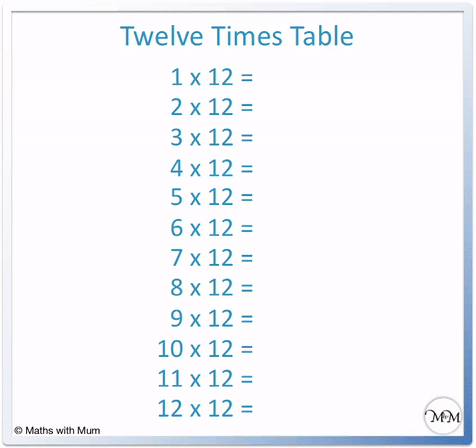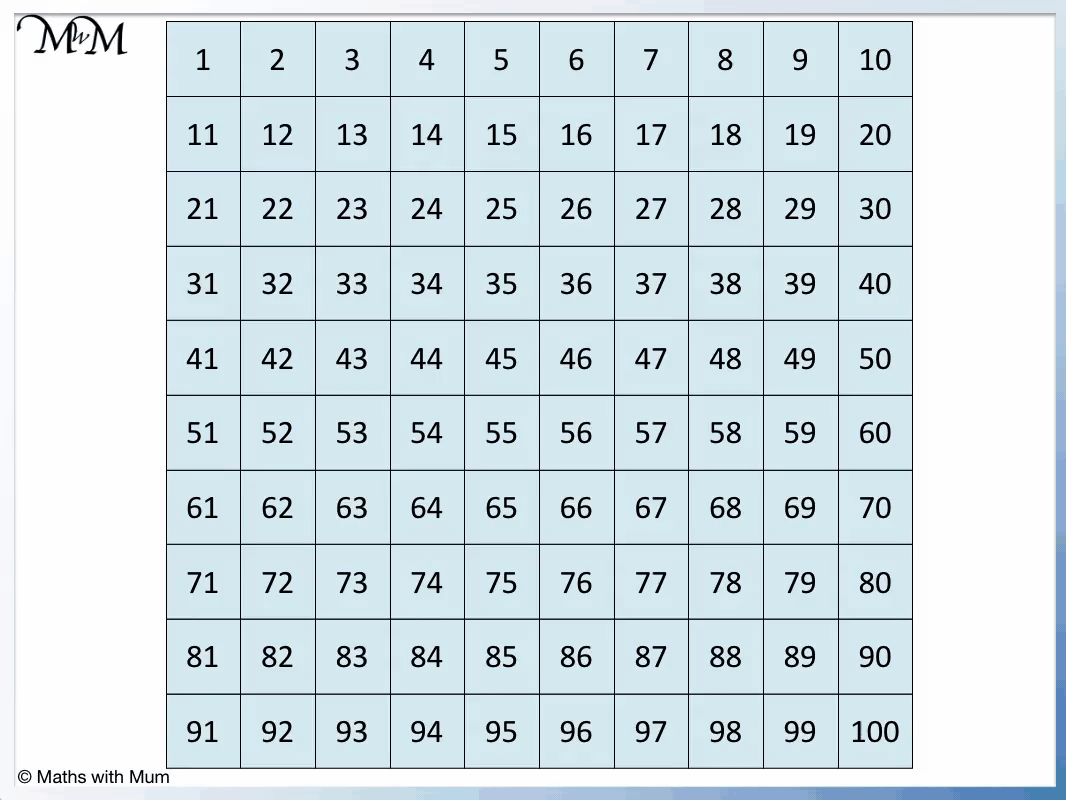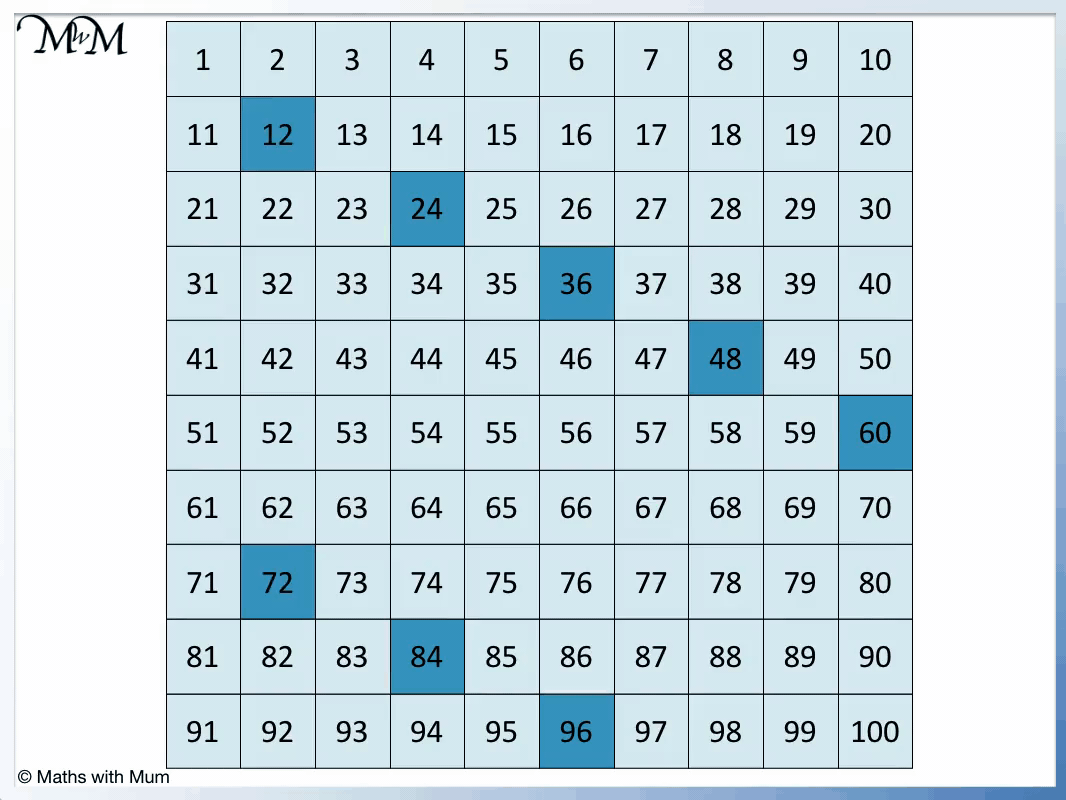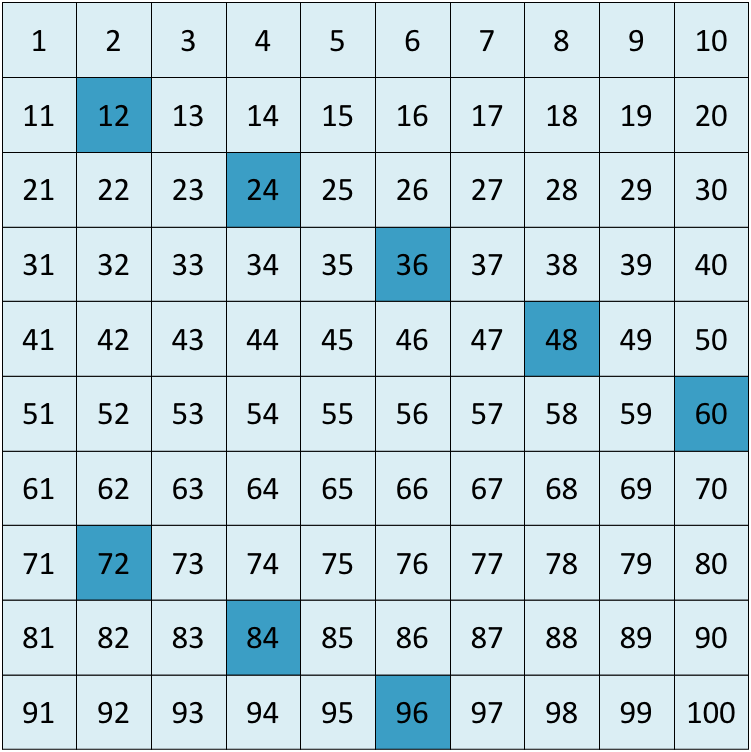# Multiples of 12

Multiples of 12• The multiples of 12 are numbers that can be divided exactly by 12, leaving no remainder.
• The first few multiples of 12 are the numbers in the 12 times table.
• For example 2 × 12 = 24 and so, the second multiple of twelve is 24.
• For example 100 × 12 = 1200 and so, the hundredth multiple of 12 is 1200.

A multiple of 12 is any number that can be divided by 12 exactly, leaving no remainder.#### Multiples of 12 Flashcards

Click on the multiples of 12 flashcards below to memorise the 12 times table:

×
×

=#### Multiples of 12: Interactive Questions

Multiples of 12: Interactive Questions

# Multiples of 12

## What are Multiples of 12

Multiples of 12 are numbers that can be divided exactly by 12 with no remainder. Multiples of 12 are the numbers formed when any whole number is multiplied by 12. For example, the third multiple of 12 is 36 because 12 × 3 = 36.

The first multiples of 12 are found in the twelve times tables. The first multiples of 12 are shown in the following poster.The first multiples of 12 are:

• 1 × 12 = 12
• 2 × 12 = 24
• 3 × 12 = 36
• 4 × 12 = 48
• 5 × 12 = 60
• 6 × 12 = 72
• 7 × 12 = 84
• 8 × 12 = 96
• 9 × 12 = 108
• 10 × 12 = 120
• 11 × 12 = 132
• 12 × 12 = 144

There is an infinite number of multiples of 12. This is because we can continue adding 12 to find the next one.

## How to Find the Multiples of 12

To find multiples of 12, multiply any whole number by 12. For example, the tenth multiple of 12 is 120 because 10 × 12 = 120. Alternatively, start from 0 and count up in twelves to find the multiples of 12.

Starting from 0 we can count up in twelves.

0 + 12 = 12. 12 + 12 = 24. 24 + 12 = 36. 36 + 12 = 48 and so on.

Here is a chart showing the multiples of 12 found by counting up in twelves each time.

To get from one multiple of 12 to the next, simply add 12.When teaching multiples of 12, it helps to look for patterns. We can remember that multiples of twelve repeat the pattern of ending in 2, 4, 6, 8 and 0. All multiples of 12 are even.When finding larger multiples of 12, it is quicker to simply multiply 12 by the multiple required.

To find the hundredth multiple of 12, multiply 12 by 100. 12 × 100 = 1200 and so, the hundredth multiple of 12 is 1200.

## Multiples of 12 up to 100

There are 8 multiples of 12 that are less than 100. They are:

12, 24, 36, 48, 60, 72, 84 and 96.

Here is a chart showing all of the multiples of 12 up to 100.Here are the first 100 multiples of 12:

12, 24, 36, 48, 60, 72, 84, 96, 108, 120, 132, 144, 156, 168, 180, 192, 204, 216, 228, 240, 252, 264, 276, 288, 300, 312, 324, 336, 348, 360, 372, 384, 396, 408, 420, 432, 444, 456, 468, 480, 492, 504, 516, 528, 540, 552, 564, 576, 588, 600, 612, 624, 636, 648, 660, 672, 684, 696, 708, 720, 732, 744, 756, 768, 780, 792, 804, 816, 828, 840, 852, 864, 876, 888, 900, 912, 924, 936, 948, 960, 972, 984, 996, 1008, 1020, 1032, 1044, 1056, 1068, 1080, 1092, 1104, 1116, 1128, 1140, 1152, 1164, 1176, 1188 and 1200.Now try our lesson on Finding Factors of a Number where we learn how to find factors.